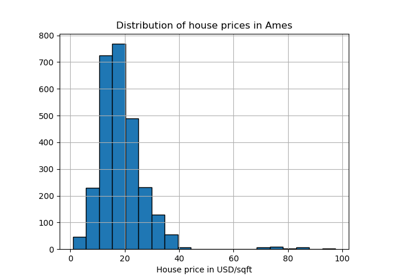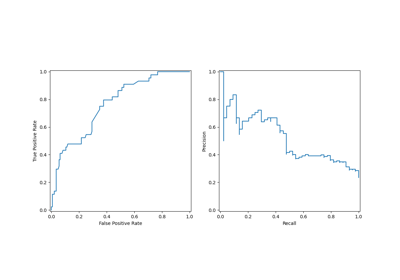# sklearn.metrics.RocCurveDisplay¶

class sklearn.metrics.RocCurveDisplay(*, fpr, tpr, roc_auc=None, estimator_name=None, pos_label=None)[source]

ROC Curve visualization.

It is recommend to use from_estimator or from_predictions to create a RocCurveDisplay. All parameters are stored as attributes.

Read more in the User Guide.

Parameters:
fprndarray

False positive rate.

tprndarray

True positive rate.

roc_aucfloat, default=None

Area under ROC curve. If None, the roc_auc score is not shown.

estimator_namestr, default=None

Name of estimator. If None, the estimator name is not shown.

pos_labelstr or int, default=None

The class considered as the positive class when computing the roc auc metrics. By default, estimators.classes_ is considered as the positive class.

New in version 0.24.

Attributes:
line_matplotlib Artist

ROC Curve.

ax_matplotlib Axes

Axes with ROC Curve.

figure_matplotlib Figure

Figure containing the curve.

roc_curve

Compute Receiver operating characteristic (ROC) curve.

RocCurveDisplay.from_estimator

Plot Receiver Operating Characteristic (ROC) curve given an estimator and some data.

RocCurveDisplay.from_predictions

Plot Receiver Operating Characteristic (ROC) curve given the true and predicted values.

roc_auc_score

Compute the area under the ROC curve.

Examples

>>> import matplotlib.pyplot as plt
>>> import numpy as np
>>> from sklearn import metrics
>>> y = np.array([0, 0, 1, 1])
>>> pred = np.array([0.1, 0.4, 0.35, 0.8])
>>> fpr, tpr, thresholds = metrics.roc_curve(y, pred)
>>> roc_auc = metrics.auc(fpr, tpr)
>>> display = metrics.RocCurveDisplay(fpr=fpr, tpr=tpr, roc_auc=roc_auc,
...                                   estimator_name='example estimator')
>>> display.plot()
<...>
>>> plt.show()


Methods

 from_estimator(estimator, X, y, *[, ...]) Create a ROC Curve display from an estimator. from_predictions(y_true, y_pred, *[, ...]) Plot ROC curve given the true and predicted values. plot([ax, name]) Plot visualization.
classmethod from_estimator(estimator, X, y, *, sample_weight=None, drop_intermediate=True, response_method='auto', pos_label=None, name=None, ax=None, **kwargs)[source]

Create a ROC Curve display from an estimator.

Parameters:
estimatorestimator instance

Fitted classifier or a fitted Pipeline in which the last estimator is a classifier.

X{array-like, sparse matrix} of shape (n_samples, n_features)

Input values.

yarray-like of shape (n_samples,)

Target values.

sample_weightarray-like of shape (n_samples,), default=None

Sample weights.

drop_intermediatebool, default=True

Whether to drop some suboptimal thresholds which would not appear on a plotted ROC curve. This is useful in order to create lighter ROC curves.

response_method{‘predict_proba’, ‘decision_function’, ‘auto’} default=’auto’

Specifies whether to use predict_proba or decision_function as the target response. If set to ‘auto’, predict_proba is tried first and if it does not exist decision_function is tried next.

pos_labelstr or int, default=None

The class considered as the positive class when computing the roc auc metrics. By default, estimators.classes_ is considered as the positive class.

namestr, default=None

Name of ROC Curve for labeling. If None, use the name of the estimator.

axmatplotlib axes, default=None

Axes object to plot on. If None, a new figure and axes is created.

**kwargsdict

Keyword arguments to be passed to matplotlib’s plot.

Returns:
displayRocCurveDisplay

The ROC Curve display.

roc_curve

Compute Receiver operating characteristic (ROC) curve.

RocCurveDisplay.from_predictions

ROC Curve visualization given the probabilities of scores of a classifier.

roc_auc_score

Compute the area under the ROC curve.

Examples

>>> import matplotlib.pyplot as plt
>>> from sklearn.datasets import make_classification
>>> from sklearn.metrics import RocCurveDisplay
>>> from sklearn.model_selection import train_test_split
>>> from sklearn.svm import SVC
>>> X, y = make_classification(random_state=0)
>>> X_train, X_test, y_train, y_test = train_test_split(
...     X, y, random_state=0)
>>> clf = SVC(random_state=0).fit(X_train, y_train)
>>> RocCurveDisplay.from_estimator(
...    clf, X_test, y_test)
<...>
>>> plt.show()

classmethod from_predictions(y_true, y_pred, *, sample_weight=None, drop_intermediate=True, pos_label=None, name=None, ax=None, **kwargs)[source]

Plot ROC curve given the true and predicted values.

Read more in the User Guide.

New in version 1.0.

Parameters:
y_truearray-like of shape (n_samples,)

True labels.

y_predarray-like of shape (n_samples,)

Target scores, can either be probability estimates of the positive class, confidence values, or non-thresholded measure of decisions (as returned by “decision_function” on some classifiers).

sample_weightarray-like of shape (n_samples,), default=None

Sample weights.

drop_intermediatebool, default=True

Whether to drop some suboptimal thresholds which would not appear on a plotted ROC curve. This is useful in order to create lighter ROC curves.

pos_labelstr or int, default=None

The label of the positive class. When pos_label=None, if y_true is in {-1, 1} or {0, 1}, pos_label is set to 1, otherwise an error will be raised.

namestr, default=None

Name of ROC curve for labeling. If None, name will be set to "Classifier".

axmatplotlib axes, default=None

Axes object to plot on. If None, a new figure and axes is created.

**kwargsdict

Additional keywords arguments passed to matplotlib plot function.

Returns:
displayRocCurveDisplay

Object that stores computed values.

roc_curve

Compute Receiver operating characteristic (ROC) curve.

RocCurveDisplay.from_estimator

ROC Curve visualization given an estimator and some data.

roc_auc_score

Compute the area under the ROC curve.

Examples

>>> import matplotlib.pyplot as plt
>>> from sklearn.datasets import make_classification
>>> from sklearn.metrics import RocCurveDisplay
>>> from sklearn.model_selection import train_test_split
>>> from sklearn.svm import SVC
>>> X, y = make_classification(random_state=0)
>>> X_train, X_test, y_train, y_test = train_test_split(
...     X, y, random_state=0)
>>> clf = SVC(random_state=0).fit(X_train, y_train)
>>> y_pred = clf.decision_function(X_test)
>>> RocCurveDisplay.from_predictions(
...    y_test, y_pred)
<...>
>>> plt.show()

plot(ax=None, *, name=None, **kwargs)[source]

Plot visualization.

Extra keyword arguments will be passed to matplotlib’s plot.

Parameters:
axmatplotlib axes, default=None

Axes object to plot on. If None, a new figure and axes is created.

namestr, default=None

Name of ROC Curve for labeling. If None, use estimator_name if not None, otherwise no labeling is shown.

**kwargsdict

Keyword arguments to be passed to matplotlib’s plot.

Returns:
displayRocCurveDisplay

Object that stores computed values.

## Examples using sklearn.metrics.RocCurveDisplay¶Evaluation of outlier detection estimators

Evaluation of outlier detection estimatorsVisualizations with Display Objects

Visualizations with Display Objects